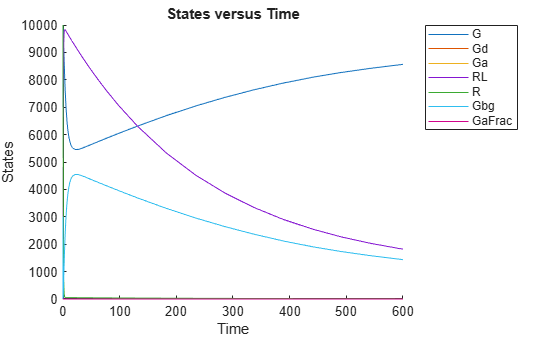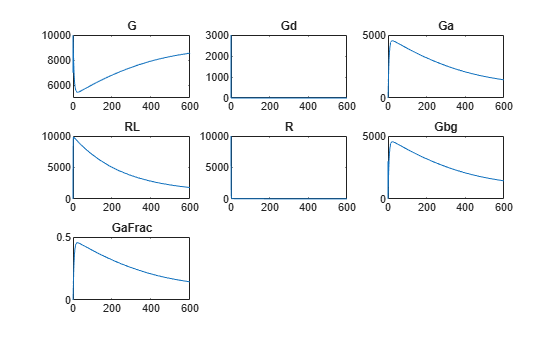# getdata

Get simulation data from `SimData` object

## Syntax

``[t,x,names] = getdata(simdata)``
``sdOut = getdata(simdata)``
``___ = getdata(simdata,format)``

## Description

example

````[t,x,names] = getdata(simdata)` returns the simulation time points `t`, the simulation data `x`, and corresponding `names` for the data columns.```
````sdOut = getdata(simdata)` returns the simulation results as a `SimData` object `sdOut`.```

example

````___ = getdata(simdata,format)` returns the simulation data in the specified `format`.```

## Examples

collapse all

`sbioloadproject('gprotein.sbproj');`

Simulate the model.

```sdObj = sbiosimulate(m1); sbioplot(sdObj);```The plot shows all the states together. Plot each state separately on its own axes in a subplot.

First, extract the simulation data from the `SimData` object.

`[time,data,names] = getdata(sdObj);`

Calculate the number of rows and columns needed for the subplot.

```sqrtnames = sqrt(numel(names)); nrows = round(sqrtnames); ncolumns = ceil(sqrtnames);```

Create a subplot and plot each state on its own axes.

```figure for(i = 1:numel(names)) subplot(nrows,ncolumns,i) plot(time,data(:,i)); title(names(i)); end```## Input Arguments

collapse all

Simulation data, specified as a `SimData` object or array of `SimData` objects.

Simulation data format, specified as a character vector or string. Some formats require you to specify only one output argument. The valid formats follow.

• `'num'` — This format returns simulation time points and simulation data in numeric arrays and the names of quantities and sensitivities as a cell array. This format is the default when you run `getdata` with multiple output arguments.

• `'nummetadata'` — This format returns a cell array of metadata structures instead of the names of quantities and sensitivities as the third output argument.

• `'numqualnames'` — This format returns qualified names in the third output argument to resolve ambiguities.

You must specify only one output argument for the following formats.

• `'simdata'` — This format returns data in a new `SimData` object or an array of `SimData` objects. This format is the default when you specify a single output argument.

• `'struct'` — This format returns a structure or structure array that contains both data and metadata.

• `'ts'` — This format returns data as a cell array.

• If `simdata` is scalar, the cell array is an m-by-1 array, where each element is a `timeseries` object. m is the number of quantities and sensitivities logged during the simulation.

• If `simdata` is not scalar, the cell array is k-by-1, where each element of the cell array is an m-by-1 cell array of `timeseries` objects. k is the size of `simdata`, and m is the number of quantities or sensitivities in each `SimData` object in `simdata`. In other words, the function returns an individual time series for each state or column and for each `SimData` object in `simdata`.

• `'tslumped'` — This format returns the data as a cell array of `timeseries` objects, combining data from each `SimData` object into a single time series.

## Output Arguments

collapse all

Simulation time points, returned as a numeric vector or cell array. If `simdata` is scalar, `t` is an n-by-1 vector, where n is the number of time points. If `simdata` is an array of objects, `t` is a k-by-1 cell array, where k is the size of `simdata`.

Simulation data, returned as a numeric matrix or cell array. If `simdata` is scalar, `x` is an n-by-m matrix, where n is the number of time points and m is the number of quantities and sensitivities logged during the simulation. If `simdata` is an array of objects, `x` is a k-by-1 cell array, where k is the size of `simdata`.

Names of quantities and sensitivities logged during the simulation, returned as a cell array. If `simdata` is scalar, `names` is an m-by-1 cell array. If `simdata` is an array of objects, `names` is a k-by-1 cell array, where k is the size of `simdata`.

Simulation results, returned as a `SimData` object.